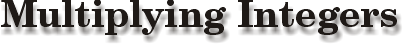##Positive × positive = positive Example: 9 × 8 = 72 Negative × negative = positive Example: (-12)(-11) = 132 Positive × negative = negative Example: (15)(-5) = -75 Example: 5 times Picksburg’s running back, "the Trolley," was stopped for a loss of 2 yards. How many yards did he lose? Answer: -10Negative × positive = negative Example: (-4)(11) = -44 Example: The Minnesota State golf team has 4 golfers that are each –6 (or 6 strokes under par) on their rounds for the day. What is the team score? Answer: -24 If there are an odd number of negative signs the answer is negative. Example: (-5)(-2)(-7) = -70 If there are an even number of negative signs the answer is positive.   Example: (5)(-2)(-7) = 70

Integer Main | Interactive Multiplication Problem in "Quest for a Cubicle"

 artist: Zach Sterba writer: Kevin Gallagher